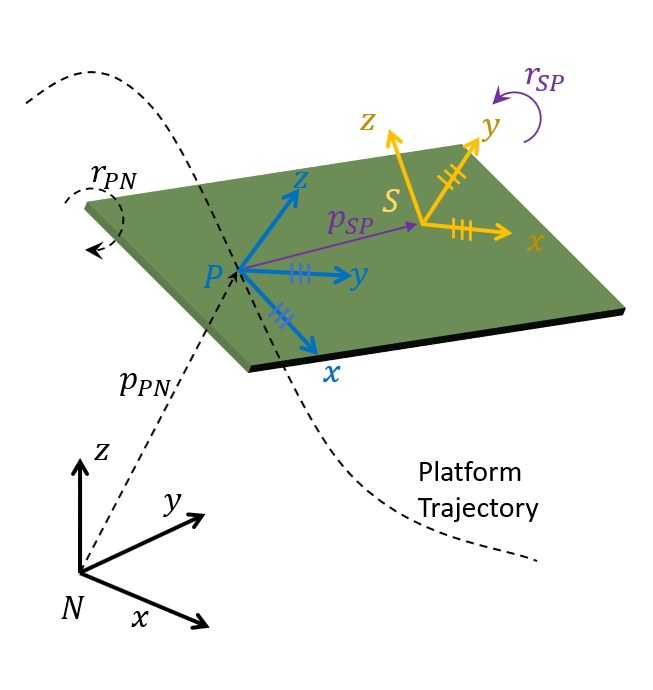# transformMotion

Compute motion quantities between two relatively fixed frames

Since R2020a

## Syntax

``[posS,orientS,velS,accS,angvelS] = transformMotion(posSFromP,orientSFromP,posP)``
``[___] = transformMotion(posSFromP,orientSFromP,posP,orientP)``
``[___] = transformMotion(posSFromP,orientSFromP,posP,orientP,velP)``
``[___] = transformMotion(posSFromP,orientSFromP,posP,orientP,velP,accP)``
``[___] = transformMotion(posSFromP,orientSFromP,posP,orientP,velP,accP,angvelP)``

## Description

````[posS,orientS,velS,accS,angvelS] = transformMotion(posSFromP,orientSFromP,posP)` computes motion quantities of the sensor frame relative to the navigation frame (`posS`, `orientS`, `velS`, `accS`, and `angvelS`) using the position of sensor frame relative to the platform frame, `posSFromP`, the orientation of the sensor frame relative to the platform frame, `orientSFromP`, and the position of the platform frame relative to the navigation frame, `posP`. Note that the position and orientation between the sensor frame and the platform frame are assumed to be fixed. Also, the unspecified quantities between the navigation frame and the platform frame (such as orientation, velocity, and acceleration) are assumed to be zero.```
````[___] = transformMotion(posSFromP,orientSFromP,posP,orientP)` additionally specifies the orientation of the platform frame relative to the navigation frame, `orientP`. The output arguments are the same as those of the previous syntax.```

example

````[___] = transformMotion(posSFromP,orientSFromP,posP,orientP,velP)` additionally specifies the velocity of the platform frame relative to the navigation frame, `velP`. The output arguments are the same as those of the previous syntax.`[___] = transformMotion(posSFromP,orientSFromP,posP,orientP,velP,accP)` additionally specifies the acceleration of the platform frame relative to the navigation frame, `accP`. The output arguments are the same as those of the previous syntax.`[___] = transformMotion(posSFromP,orientSFromP,posP,orientP,velP,accP,angvelP)` additionally specifies the angular velocity of the platform frame relative to the navigation frame, `angvelP`. The output arguments are the same as those of the previous syntax.```

## Examples

collapse all

Define the pose, velocity, and acceleration of the platform frame relative to the navigation frame.

```posPlat = [20 -1 0]; orientPlat = quaternion(1, 0, 0, 0); velPlat = [0 0 0]; accPlat = [0 0 0]; angvelPlat = [0 0 1];```

Define the position and orientation offset of IMU sensor frame relative to the platform frame.

```posPlat2IMU = [1 2 3]; orientPlat2IMU = quaternion([45 0 0], 'eulerd', 'ZYX', 'frame');```

Calculate the motion quantities of the sensor frame relative to the navigation frame and print the results.

```[posIMU, orientIMU, velIMU, accIMU, angvelIMU] ... = transformMotion(posPlat2IMU, orientPlat2IMU, ... posPlat, orientPlat, velPlat, accPlat, angvelPlat); fprintf('IMU position is:\n');```
```IMU position is: ```
`fprintf('%.2f %.2f %.2f\n', posIMU);`
```21.00 1.00 3.00 ```
`orientIMU`
```orientIMU = quaternion 0.92388 + 0i + 0j + 0.38268k ```
`velIMU`
```velIMU = 1×3 -2 1 0 ```
`accPlat`
```accPlat = 1×3 0 0 0 ```

## Input Arguments

collapse all

Position of the sensor frame relative to the platform frame, specified as a 1-by-3 vector of real scalars.

Example: `[1 2 3]`

Orientation of the sensor frame relative to the platform frame, specified as a `quaternion` or a 3-by-3 rotation matrix.

Example: `quaternion(1,0,0,0)`

Position of platform frame relative to navigation frame, specified as an N-by-3 matrix of real scalars. N is the number of position quantities.

Example: `[1 2 3]`

Orientation of platform frame relative to navigation frame, specified as an N-by-1 array of quaternions, or a 3-by-3-by-N array of scalars. Each 3-by-3 matrix must be a rotation matrix. N is the number of orientation quantities.

Example: `quaternion(1,0,0,0)`

Velocity of platform frame relative to navigation frame, specified as an N-by-3 matrix of real scalars. N is the number of velocity quantities.

Example: `[ 4 8 6]`

Acceleration of platform frame relative to navigation frame, specified as an N-by-3 matrix of real scalars. N is the number of acceleration quantities.

Example: `[4 8 6]`

Angular velocity of platform frame relative to navigation frame, specified as an N-by-3 matrix of real scalars. N is the number of angular velocity quantities.

Example: `[4 2 3]`

## Output Arguments

collapse all

Position of sensor frame relative to navigation frame, returned as an N-by-3 matrix of real scalars. N is the number of position quantities specified by the `posP` input.

Orientation of sensor frame relative to navigation frame, returned as an N-by-1 array of quaternions, or a 3-by-3-by-N array of scalars. N is the number of orientation quantities specified by the `orientP` input. The returned orientation quantity type is same with the `orientP` input.

Velocity of sensor frame relative to navigation frame, returned as an N-by-3 matrix of real scalars. N is the number of position quantities specified by the `velP` input.

Acceleration of sensor frame relative to navigation frame, returned as an N-by-3 matrix of real scalars. N is the number of position quantities specified by the `accP` input.

Angular velocity of sensor frame relative to navigation frame, returned as an N-by-3 matrix of real scalars. N is the number of position quantities specified by the `angvelP` input.

collapse all

### Motion Quantities Used in `transformMotion`

The `transformMotion` function calculates the motion quantities of the sensor frame (S), which is fixed on a rigid platform, relative to the navigation frame (N) using the mounting information of the sensor on the platform and the motion information of the platform frame (P).

As shown in the figure, the position and orientation of the platform frame and the sensor frame are fixed on the platform. The position of the sensor frame relative to the platform frame is pSP, and the orientation of the sensor frame relative to the platform frame is rSP. Since the two frames are both fixed, pSP and rSP are constant.

To compute the motion quantities of the sensor frame relative to the navigation frame, the quantities describing the motion of the platform frame relative to the navigation frame are required. These quantities include: the platform position (pPN), orientation (rPN), velocity, acceleration, angular velocity, and angular acceleration relative to the navigation frame. You can specify these quantities through the function input arguments except the angular acceleration, which is always assumed to be zero in the function. The unspecified quantities are also assumed to be zero.## Version History

Introduced in R2020a